# Doctor of Philosophy

N/A
N/A
Protected

Share "Doctor of Philosophy"

Copied!
165
0
0

Full text

First, a test of the new physical sensitivities of the various observers associated with these decays in future high-luminosity experiments. In addition, the best-fit values ​​and correlations of the new parameters are extracted using the Akaike Information Criterion in the selected scenarios.

## Flavor in the standard model

Because the mass matrices are proportional to the Yukawa matrices, the Yukawa interactions in the mass basis are diagonal. The neutral current part of the lagrangian in the mass basis remains unchanged, that is, in SM the flavor-changing neutral currents (FCNC) are not allowed at the tree level.

### Unitarity Triangle

The length of the sides of the UT can be identified in terms of these parameters as follows:.

### Determination of CKM parameters

The UT angles α, β, γ mentioned in the left panel of Table 1.2 can be determined experimentally from CP-violating measurements with less theoretical uncertainty (≤ . 10%). These semileptonic decays, being at the tree level, the lowest order in the SM, are expected to be free from any new physical effects, thus providing a clean environment for measuring Vcb.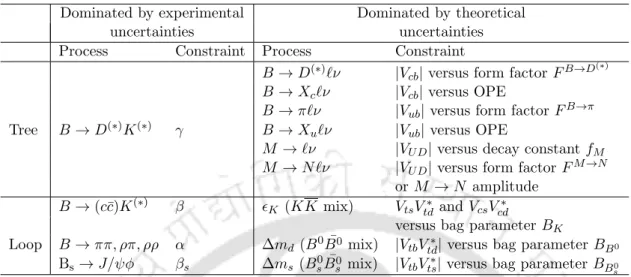Table 1.2: A partial list of measurements generally used to determine the CKM param- param-eters

## New Physics and the Observables

### New Operators

In the framework of effective theory, one can write the most general effective new operators, which may include new scalar, vector and tensor currents, other than those in SM, to analyze these b→cτ ντ decays. The most general effective Hamiltonian describing b→c`ν` transitions (where `=e, µorτ) with all possible four-fermion operators in the lowest dimension is given by .

### Observables

• B → D (∗) τ ν τ
• B c → J/ψ ` ν `
• Λ b → Λ c `ν `
• B → X c τ ν ¯ τ
• B c → τ ν τ

These helicity amplitudes are related to the shape factors as follows (f+ andf0 forB →Dan and V,A0,A1 and A2 forB →D∗). As mentioned earlier, the hadronic form factors V1(w) and S1(w) coincide with the Isgur-Wise function ξ(w) in the infinite mass limit of the heavy quark mQ ( = mb or mc).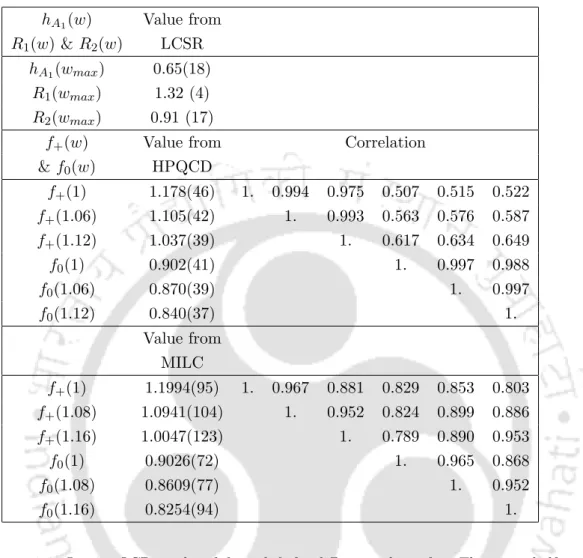Table 1.3: Lattice QCD results of f + and f 0 for different values of w. The upper half of the table have been obtained using the fit results from the HPQCD collaboration , and the lower half are the results obtained by the Fermilab MILC collaboration

## Experimental status

The Bc→τ ν decay, although so far out of experimental reach , can be used as an effective constraint on any NP effects that could potentially explain the excesses of RD(∗) and RJ/ψ. From the discussion presented in the previous section, it is now clear to us that there are several strong lines of evidence and hints that motivate us to consider BSM scenarios in b→ cτ ντ decays to explain the observed deviations of the SM predictions of several observables from their experimental results .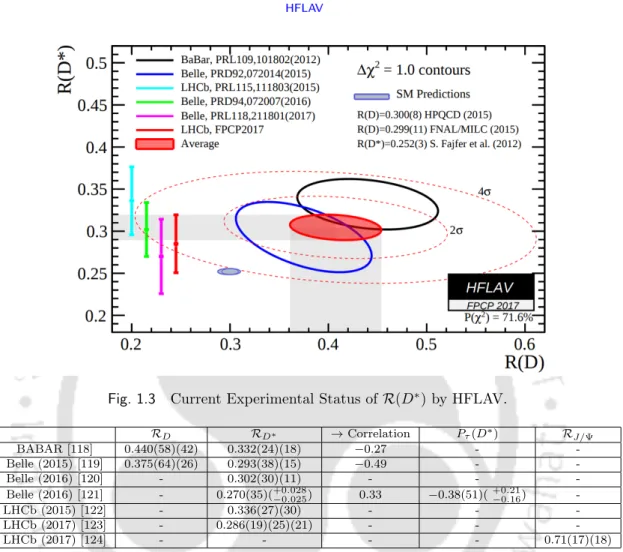Fig. 1.3 Current Experimental Status of R(D ∗ ) by HFLAV.

## Optimal Observable Technique

This technique allows one to systematically estimate the statistical uncertainty of the measurable parameters while extracting it from a number of observable parameters. In the remainder of this chapter we will provide a detailed discussion of the methodology of these two analyses. This meaning depends, as we must emphasize here, on the chosen observable, on the parameters of the NP model and on the integrated luminosity, all of which are intuitively obvious.

## Goodness-of-Fit

The mandatory step to assess the suitability of an analysis after optimization is then to inspect the distribution of the residuals. The comparison of the residuals with this Gaussian can be objectively measured by the Shapiro-Wilks (S-W) test  for normality. In all fitted models, those with a p-value of the residual distribution above 5% will be considered to fit the data well; all the rest can be thrown out.

## Model Selection

### Introduction to AIC c

Often the number of parameters in a model is used as a measure of the degree of structure inferred from the data. In the application, AIC is calculated for each of the candidate models and the model with the smallest value of AIC is selected. This can be done by extending the concept of the probability of the parameters given both the data and the model, i.e.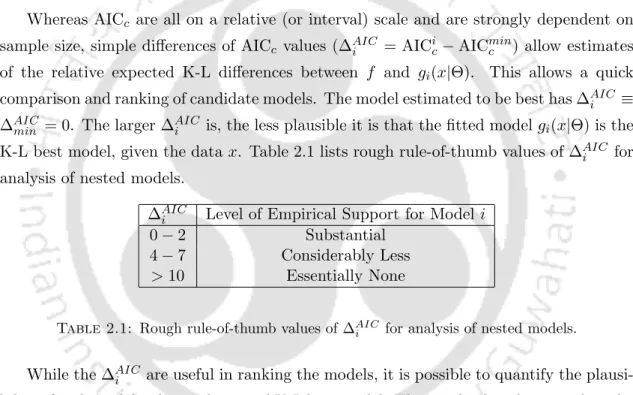Table 2.1: Rough rule-of-thumb values of ∆ AIC i for analysis of nested models.

## Introduction

In section 3.2 we first discuss the formalism of the optimal observable technique in the context of several observables in B →D(∗)τ ντ decay channels. A numerical analysis is presented in section 3.3 that tests the new physics sensitivity of each of the integrated observables considered in this study. In the next part of section 3.3, we performed the bin-by-bin analysis of q2 distribution of the differential branching fraction for B → D(∗)τ ντ decay to zoom into the regions of q2 where a specific type NP interaction shows maximum sensitivity to the considered observable.

## Formalism

The coefficients Ci, relevant for the branching fractions in B → D(∗)τ ντ, are given in Table 3.1, and the corresponding fi(q2)s are given in the Appendix (Table C.1). However, there is a unique choice for which the resulting error in deriving Ci is minimized1, and these functions are given by. As given in equation (3.4), the errors are also related to the output cross section σP ( = σB→D(∗)τ ντ/B(B →D(∗)τ ντ)), and the effective brightness Lef f = Lints, where Linta and s are the integrated brightness and reconstruction efficiency, respectively. As we know that the cross section σa→b =σaΓb/Γ, therefore, we can define σB→D(∗)τ ντ =σPB(B → D(∗)τ . ντ), where σP is the product cross section BB¯.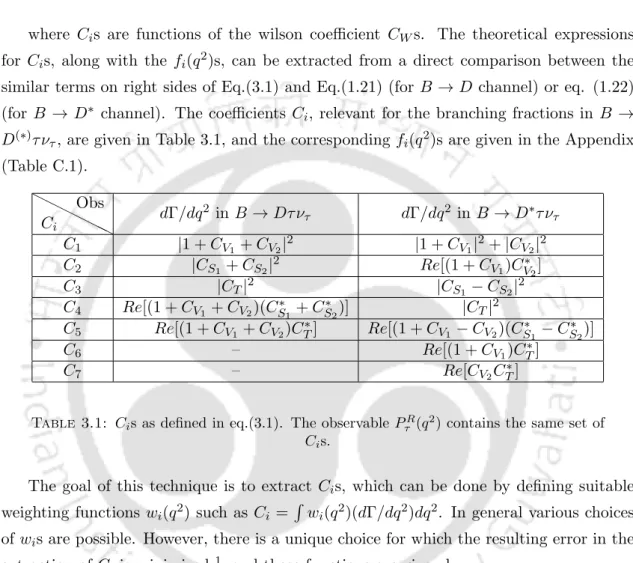Table 3.1: C i s as defined in eq.(3.1). The observable P τ R (q 2 ) contains the same set of C i s.

## Analysis

### Discussions

It is also important to note that this observer is more sensitive to the real part of the coefficients than to the imaginary part. The forward–backward asymmetry in B → Dτ ντ is equally sensitive to scalar- and tensor-type interactions. where C1 and C2 are functions of the Wilson coefficients of the vector operators. On the other hand, D∗ polarization is equally sensitive to scalar and tensor interactions.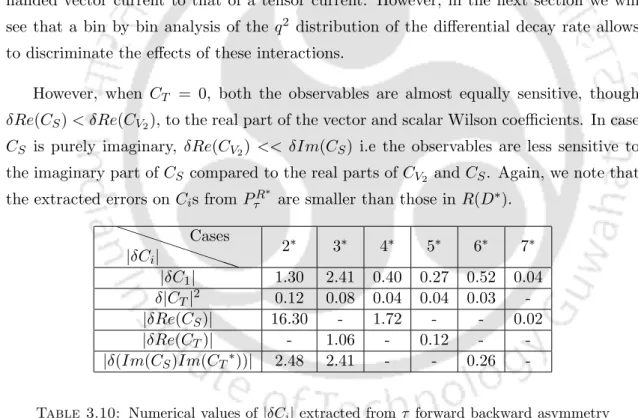Table 3.10: Numerical values of |δC i | extracted from τ forward backward asymmetry in B → D ∗ τ ν τ .

### Bin-by-bin analysis

We note that in the low q2 region (<~7GeV2/c4) the differential decay rate is sensitive to the scalar interaction 3, the sensitivity to tensor interaction in this region is very weak, whereas in the highq2 region (>- 7GeV2/c4) ) it is quite sensitive to the tensor interaction. On the other hand, if we only see deviations in the low q2 (<7) bins, it may be due to a new scalar interaction (Fig. 3.4c). In Tables 3.12 and 3.13 we consider a few cases and give a rough estimate of the uncertainty due to the errors in fi(q2).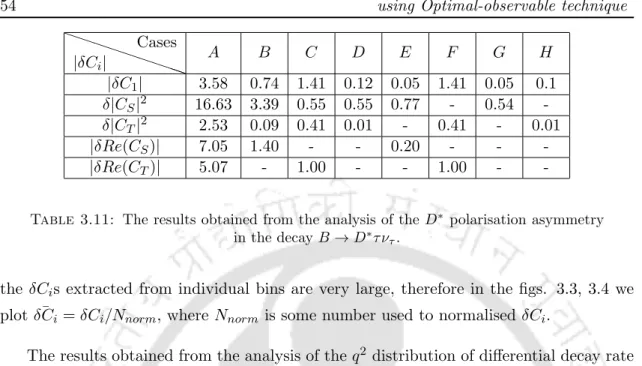Table 3.11: The results obtained from the analysis of the D ∗ polarisation asymmetry in the decay B → D ∗ τ ν τ .

## Summary

The output of the fit is used to predict the various observables associated with B →D(∗)τ ντ decays in the SM. In this part of the analysis we have not added any new physical contributions to the decay of b → cτ ντ. In another part of the analysis (section 4.3) we considered the contributions of various NP interactions in B → D(∗)τ ντ, but not in B → D(∗)`ν`.

Formalism

Since we do not have any information about the systematic uncertainties and their correlations on the pooled data, we perform two separate analyses. The second analysis is made under the assumption that the systematic uncertainties are equal to the statistical ones and a 100% systematic correlation, i.e. Vijsyst = δRexpi δRexpj and Vijstat defined as before. The utility of treating systematic uncertainties as equal to statistical ones and considering 100% systematic correlations in the second analysis is multifaceted.

### Fit Results

The analysis of the BABARbin data on R(D∗) from both 'Fit-1' and 'Fit-2' shows that the fitted parameters such asρ2D∗ and R1(1) are consistent within 2σ, with HFAG. After analyzing Belle's data on R(D∗) from 'Fit-1', we obtain large errors on ρ2D∗ and R1(1), and these are consistent with the fitted value of HFAG at 1σ. While the analysis of R(D∗) from 'Fit-1' results obtained using BABAR and Belle-binned data (Table 4.2) are roughly consistent with each other, including the best-fit values ​​of R0(1), the same analysis from 'Fit-2' (table 4.4) actually makes the results compatible.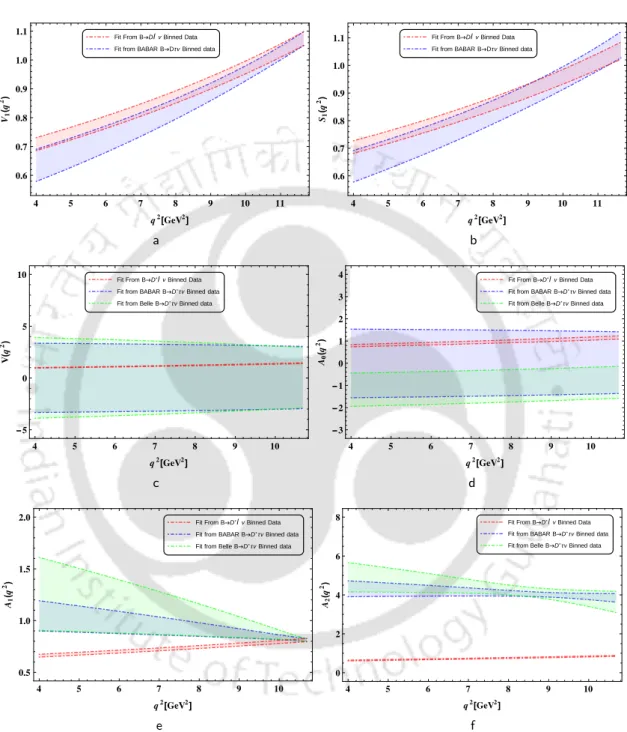Fig. 4.2 Results obtained from ‘Fit-1’. Fig.s 4.2a and 4.2b are the q 2 dependence of form-factors for semileptonic b → c transitions

## New Physics Analysis

### Methodology

• Numerical Multi-parameter Optimization

For the analysis of any specific NP model, the uncertainty of the HQET hadronic form factors and the quark masses (Table 4.6) are taken into account in the calculation of Vijth. The next column shows the results of the S-W normality test for goodness-of-fit assessment. We define the χ2 statistic for each of the 31 cases, a function of the NP Wilson coefficients.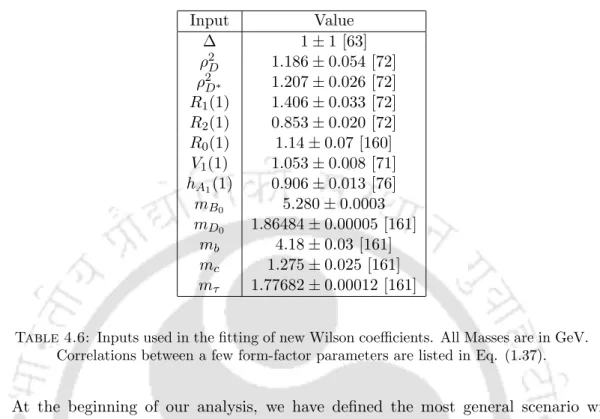Table 4.6: Inputs used in the fitting of new Wilson coefficients. All Masses are in GeV.

### Results

• Fit-1
• Fit-2

Only those NP scenarios that pass the normality test are listed in Table 4.8 with the best-fit values ​​and 1σ uncertainties of their parameters. In addition to the combined data, we performed an analysis taking into account the Bella and LHCb measurements of the integrated R(D(∗)) q2 (numerical values ​​see Table 4.1). The best-fit values ​​of the fitted parameters along with the corresponding errors are shown in Table 4.11.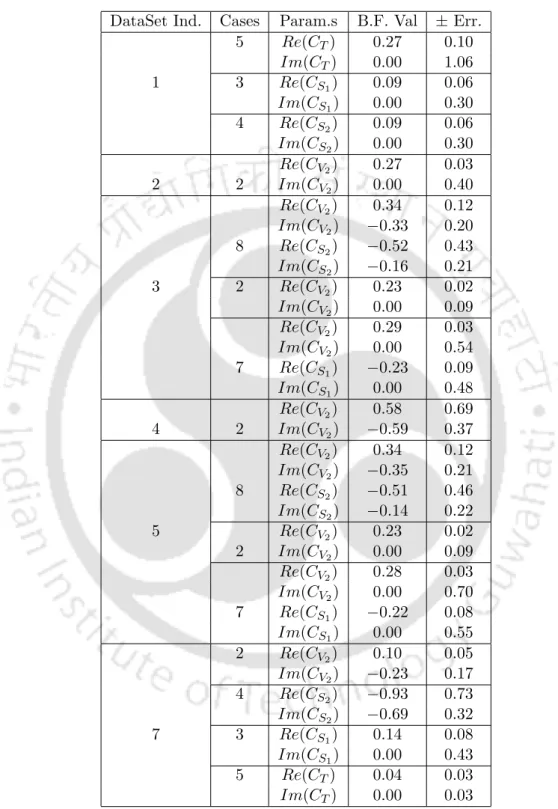Table 4.8: Best-fit values and Gaussian errors of all parameters for the selected ‘best’

## Summary

In this chapter we performed a model-independent analysis of the NP affecting different decay modes of b → cτ ντ. We also found the best-fitting values ​​of the model parameters in the selected cases. Before starting the new physics analysis, we also predicted the SM values ​​of the asymmetric and angular observables in B →D(∗)τ ντ decay, using the results of the new, current analysis in B → D(∗ )`ν`.

Exclusive Decay Channels

### Inclusive Decay

These predictions differ from each other due to the difference in the mass of the charm quark in two different schemes. For mkinc (1 GeV), the correlation matrix for the non-perturbative parameters and masses is given in Table 5.2, which is obtained from the analysis of . This is due to the difference in the correlation matrix of parameters listed in Table 5.3.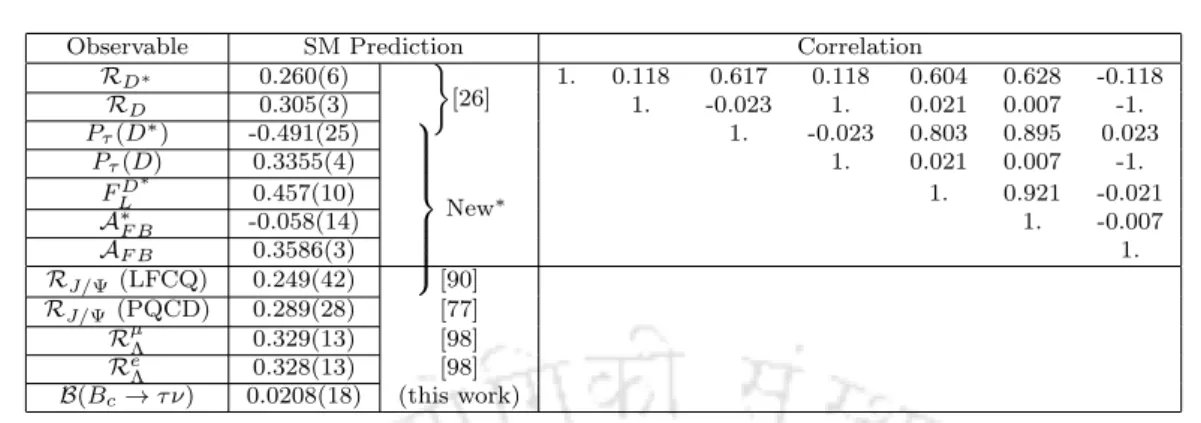Table 5.1: SM values of observables obtained and/or used in this chapter, with cor- cor-relations, wherever relevant

## New Physics Analysis

### Numerical Optimization and Model Selection

Other relevant inputs are taken from Table 5.2 in the kinetic scheme, while those for the MS scheme are taken from Table II of ref. The nuisance parameters that occur in the theoretical expressions (Table 5.8) are matched to the fit using a term. We also check the quality of the fit and the existence of outliers in the fitted data set by a 'Pull' (= Oiexp−Oith(CWk.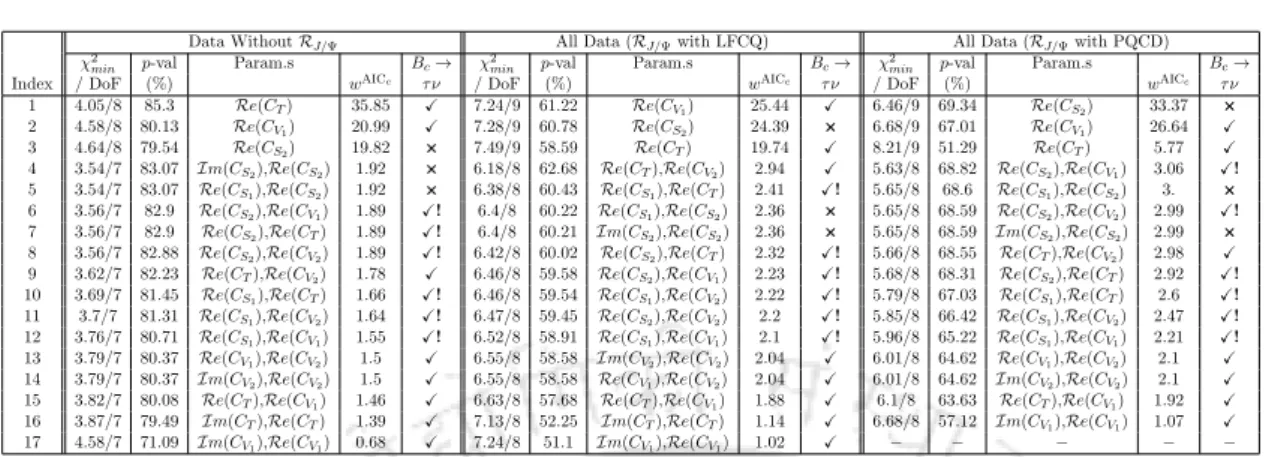Table 5.4: Scenarios selected after passing the normality check and the criterion

## Results

### Model selection

As a representative case, we have shown the plots for all scenarios listed in the last data set in Table 5.4 ('All data', where the theoretical value of RJ/Ψ is calculated in PQCD) in Figures 5.1 and 5.2. 1 As an example, for scenario 4 given in the last data set in Table 5.4 (withRe(CS2) andRe(CV1)), the symbol for the constraint column is 'X!'. In Table 5.9 (last data set) the corresponding result is quoted for the closest to zero.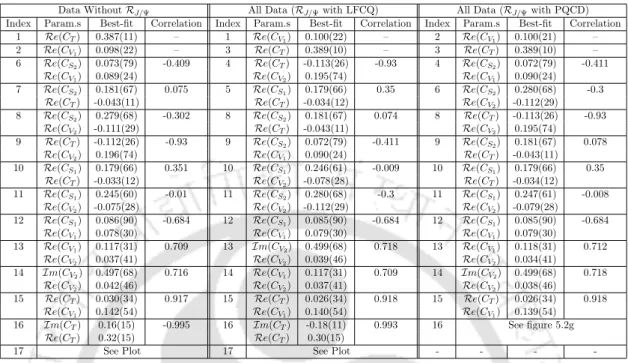Table 5.9: Best fit results and correlations of the scenarios listed in table 5.4. We omit the scenarios disallowed by the constraint B(B c → τ ν τ ) ≤ 30%

### Prediction of observables and correlations amongst them

In the presence of Re(CV1), Re(CV2), or Re(CS1), if one of the observables is consistent with the SM, then the other must be as well. However, in the case of Re(CT), there are regions where RD∗ is consistent with the SM, while RD largely deviates from its SM prediction. Due to the large uncertainty in the SM prediction ofRJ/ψ, the predicted values ​​of these observables are consistent with the SM prediction in.Fig. 5.3 Correlation plots among different observables for one-operator scenarios listed in the first column of Table 5.9

## Summary

In the absence of BABAR onRD(∗) data from our analysis, single-operator scenarios such as (V±A), S−P and tensor operators with realCW are most favored. In the absence of BABAR onR(D∗) data from our analysis, single-operator scenarios such as (V ±A), S−P and tensor operators with real CW are most favored. In our recent work, we have also predicted the SM values ​​of asymmetric and angular observers in B → D(∗)τ ντ decays using the results of the updated new analysis in B → D(∗)`ν` .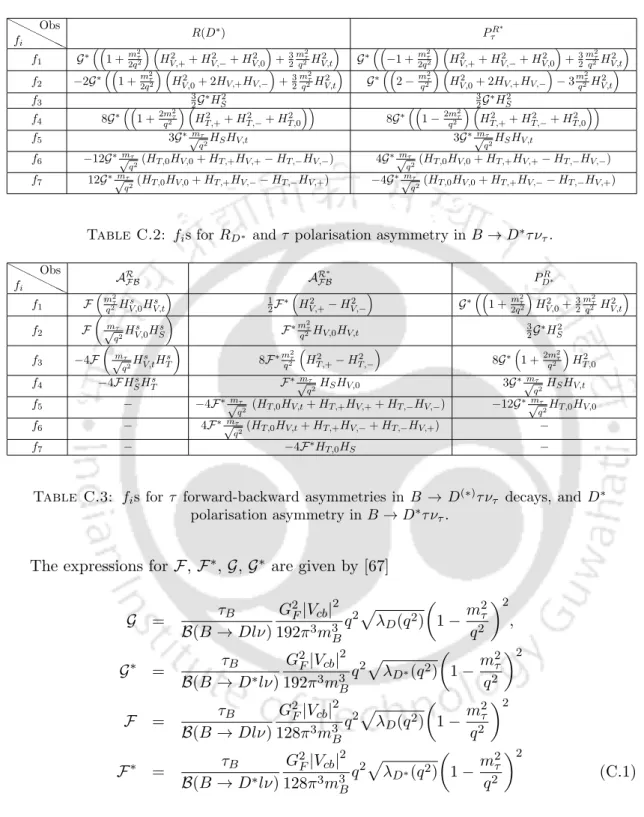Table C.2: f i s for R D ∗ and τ polarisation asymmetry in B → D ∗ τ ν τ .

Figure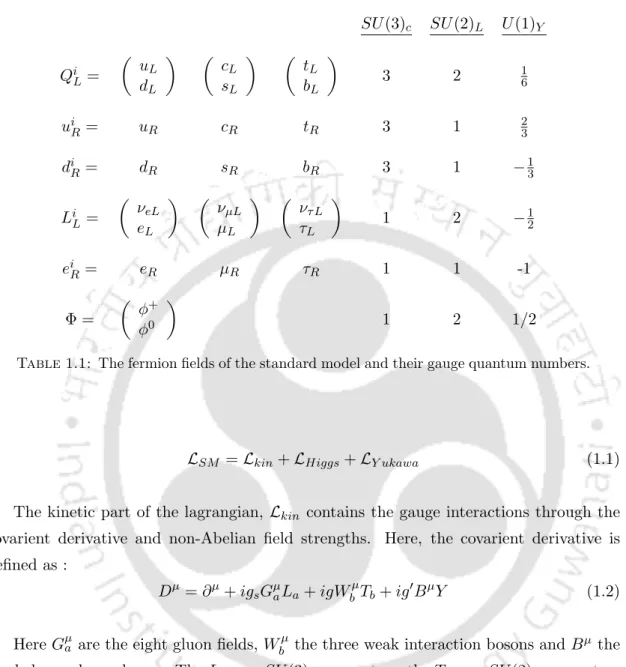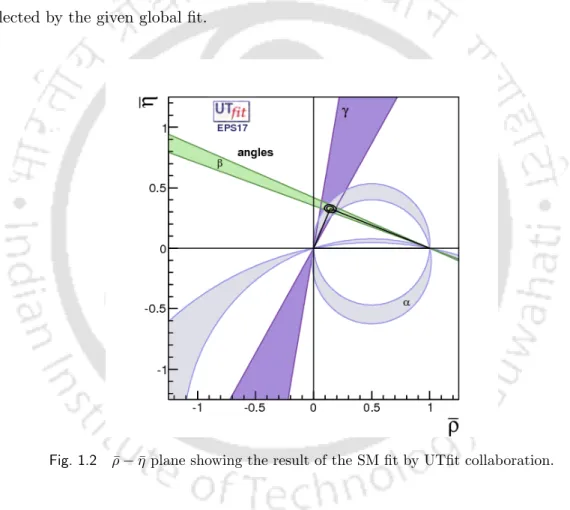+7

References

Related documents

[r]

i After successful completion of the course work, a candidate shall submit a synopsis of the proposed research work in triplicate to the Research Advisory Committee concerned through

KONDAREDLA MOUKHIKA SAHITYAM BHASHAA SAAMAAJIKA PARISHIILANA The thesis submitted to the University of Hyderabad in partial fulfillment of the degree of Doctor of Philosophy in

Rathnakar UP, Ganesh A, Unnikrishnan B, Srikanth D, Ganesh K, Shenoy A.K, Kamath A, Doctor Patient Relationship : Influence of Gender and Role.. Viewpoint of Specialist doctors in

TELANGANA STATE ELECTRICITY REGULATORY COMMISSION #11-4-660, 5th Floor, Singareni Bhavan, Red Hills, Khairatabad Hyderabad 500 004 PUBLIC NOTICE In the matter of passing

a returned to b comeback c turned up d get down Directions 61-65: In the following questions, out of the four alternatives, choose the one which best expresses the meaning of the given

Sudha, a research student in the Department of Computer Science and Engineering, Indian Institute of Technology, Guwahati, for the award of the degree of Doctor of Philosophy, is a

18 3.13 : Experimental design for continuous studies with CrVI and PANI-jute 51 19 3.14 : Experimental design for adsorption study of CrVI with support less AFC in batch mode 52 20

Following the experimental findings we argued that suffixation in Sylheti presents a case of tonal complexity where- 1 in case of noun suffixes both plural inanimate suffix and the